• Slides: 15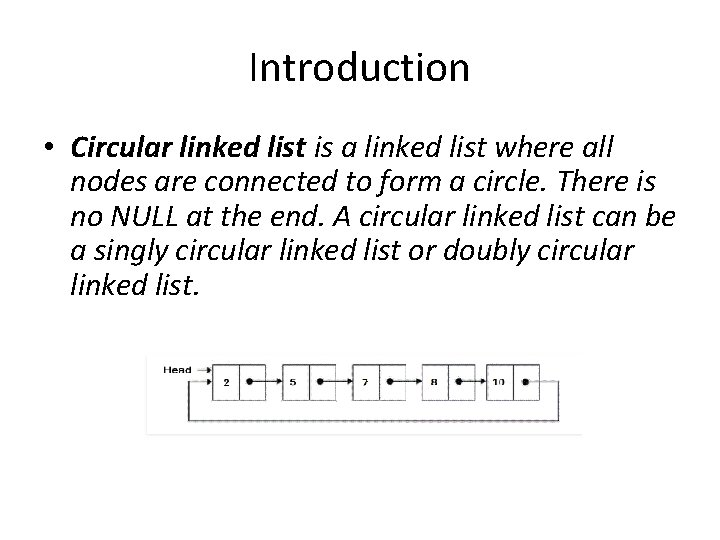Introduction • Circular linked list is a linked list where all nodes are connected to form a circle. There is no NULL at the end. A circular linked list can be a singly circular linked list or doubly circular linked list.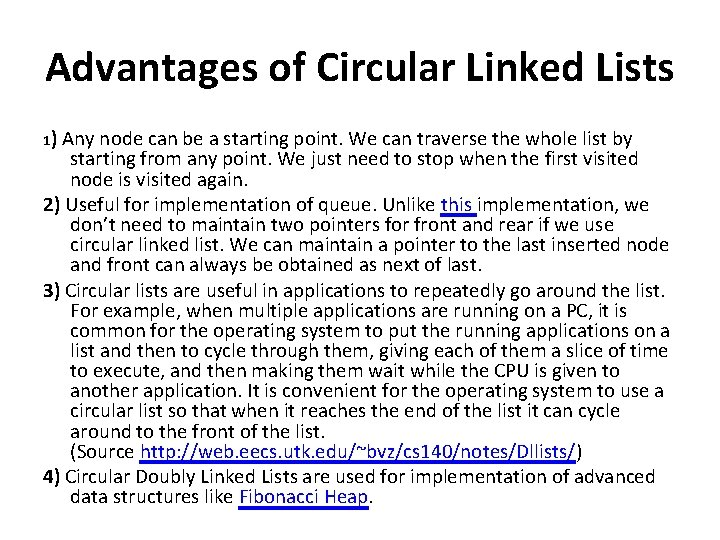Advantages of Circular Linked Lists 1) Any node can be a starting point. We can traverse the whole list by starting from any point. We just need to stop when the first visited node is visited again. 2) Useful for implementation of queue. Unlike this implementation, we don’t need to maintain two pointers for front and rear if we use circular linked list. We can maintain a pointer to the last inserted node and front can always be obtained as next of last. 3) Circular lists are useful in applications to repeatedly go around the list. For example, when multiple applications are running on a PC, it is common for the operating system to put the running applications on a list and then to cycle through them, giving each of them a slice of time to execute, and then making them wait while the CPU is given to another application. It is convenient for the operating system to use a circular list so that when it reaches the end of the list it can cycle around to the front of the list. (Source http: //web. eecs. utk. edu/~bvz/cs 140/notes/Dllists/) 4) Circular Doubly Linked Lists are used for implementation of advanced data structures like Fibonacci Heap.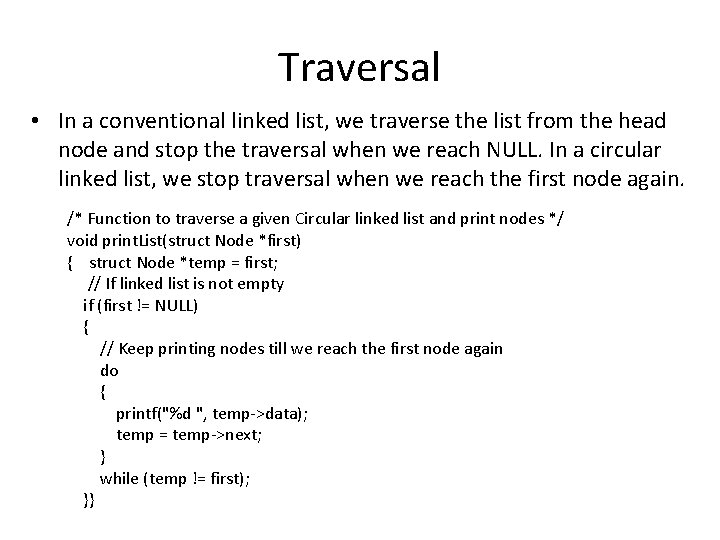Traversal • In a conventional linked list, we traverse the list from the head node and stop the traversal when we reach NULL. In a circular linked list, we stop traversal when we reach the first node again. /* Function to traverse a given Circular linked list and print nodes */ void print. List(struct Node *first) { struct Node *temp = first; // If linked list is not empty if (first != NULL) { // Keep printing nodes till we reach the first node again do { printf("%d ", temp->data); temp = temp->next; } while (temp != first); }}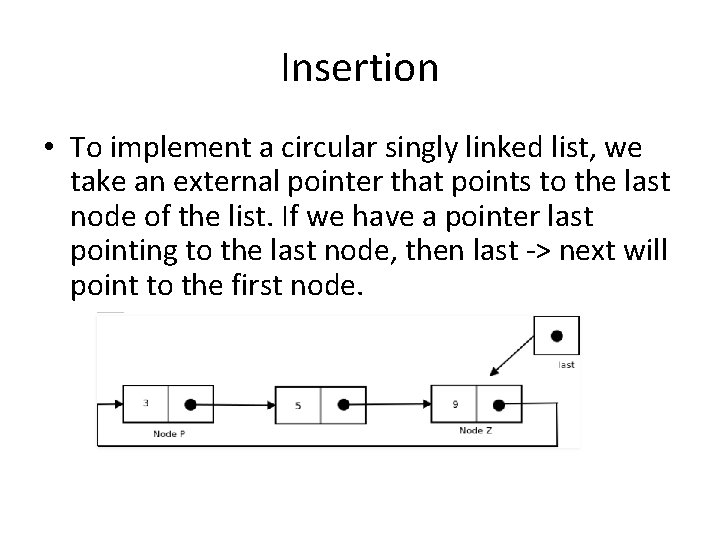Insertion • To implement a circular singly linked list, we take an external pointer that points to the last node of the list. If we have a pointer last pointing to the last node, then last -> next will point to the first node.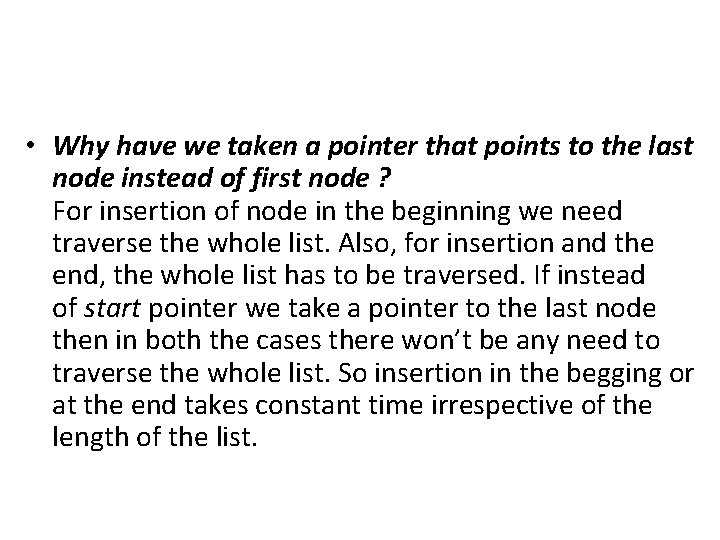• Why have we taken a pointer that points to the last node instead of first node ? For insertion of node in the beginning we need traverse the whole list. Also, for insertion and the end, the whole list has to be traversed. If instead of start pointer we take a pointer to the last node then in both the cases there won’t be any need to traverse the whole list. So insertion in the begging or at the end takes constant time irrespective of the length of the list.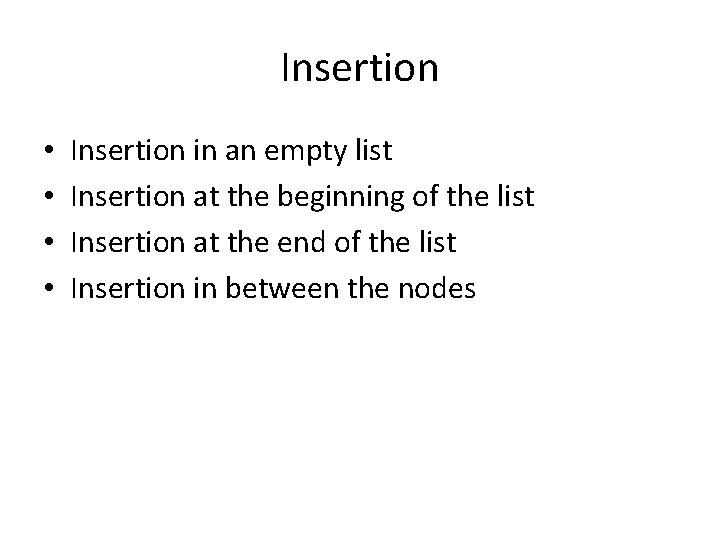Insertion • • Insertion in an empty list Insertion at the beginning of the list Insertion at the end of the list Insertion in between the nodes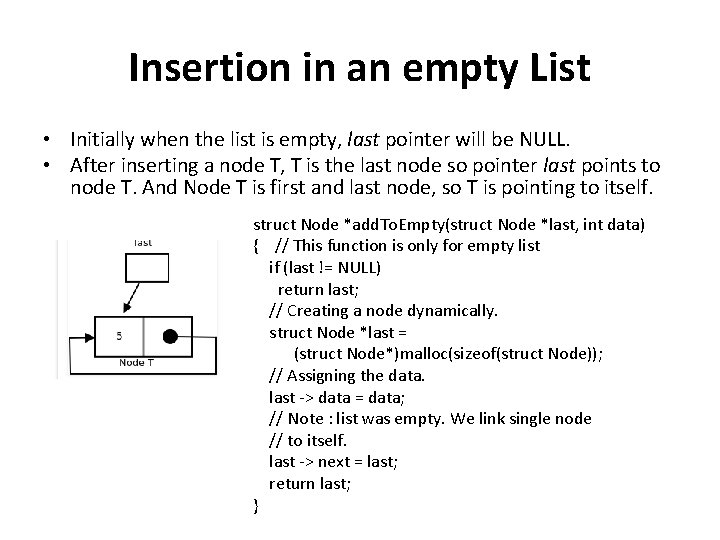Insertion in an empty List • Initially when the list is empty, last pointer will be NULL. • After inserting a node T, T is the last node so pointer last points to node T. And Node T is first and last node, so T is pointing to itself. struct Node *add. To. Empty(struct Node *last, int data) { // This function is only for empty list if (last != NULL) return last; // Creating a node dynamically. struct Node *last = (struct Node*)malloc(sizeof(struct Node)); // Assigning the data. last -> data = data; // Note : list was empty. We link single node // to itself. last -> next = last; return last; }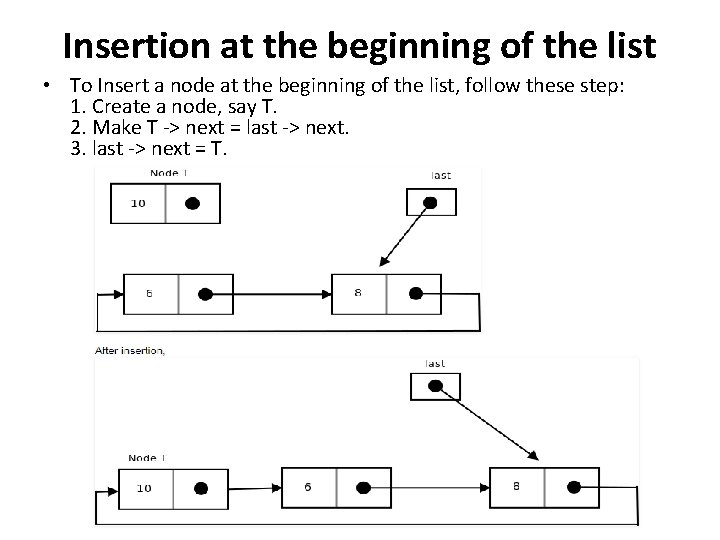Insertion at the beginning of the list • To Insert a node at the beginning of the list, follow these step: 1. Create a node, say T. 2. Make T -> next = last -> next. 3. last -> next = T.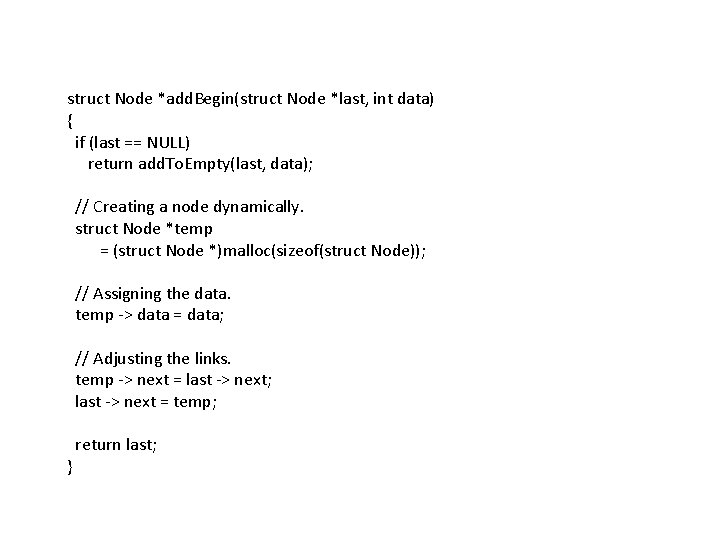struct Node *add. Begin(struct Node *last, int data) { if (last == NULL) return add. To. Empty(last, data); // Creating a node dynamically. struct Node *temp = (struct Node *)malloc(sizeof(struct Node)); // Assigning the data. temp -> data = data; // Adjusting the links. temp -> next = last -> next; last -> next = temp; return last; }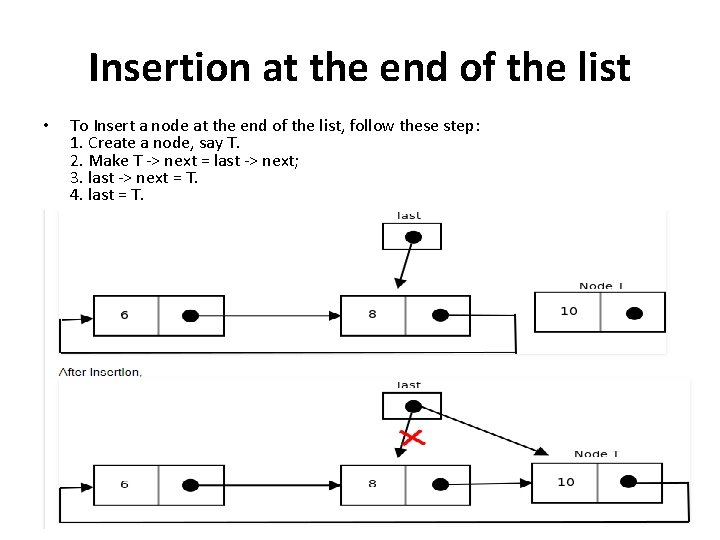Insertion at the end of the list • To Insert a node at the end of the list, follow these step: 1. Create a node, say T. 2. Make T -> next = last -> next; 3. last -> next = T. 4. last = T.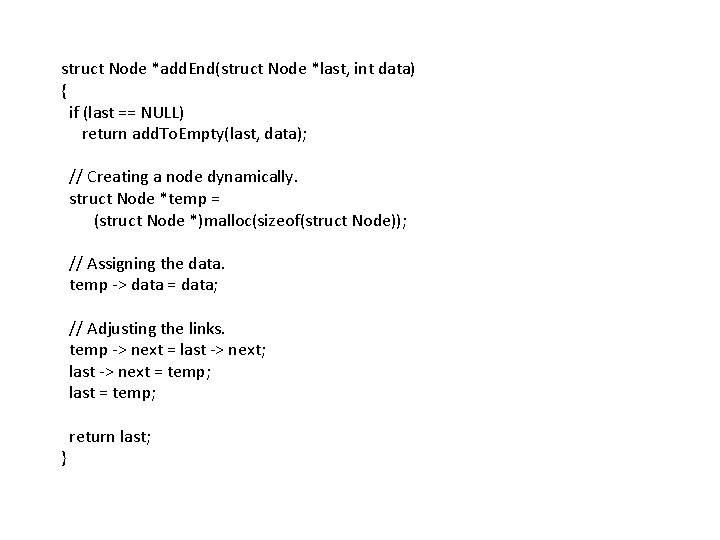struct Node *add. End(struct Node *last, int data) { if (last == NULL) return add. To. Empty(last, data); // Creating a node dynamically. struct Node *temp = (struct Node *)malloc(sizeof(struct Node)); // Assigning the data. temp -> data = data; // Adjusting the links. temp -> next = last -> next; last -> next = temp; last = temp; return last; }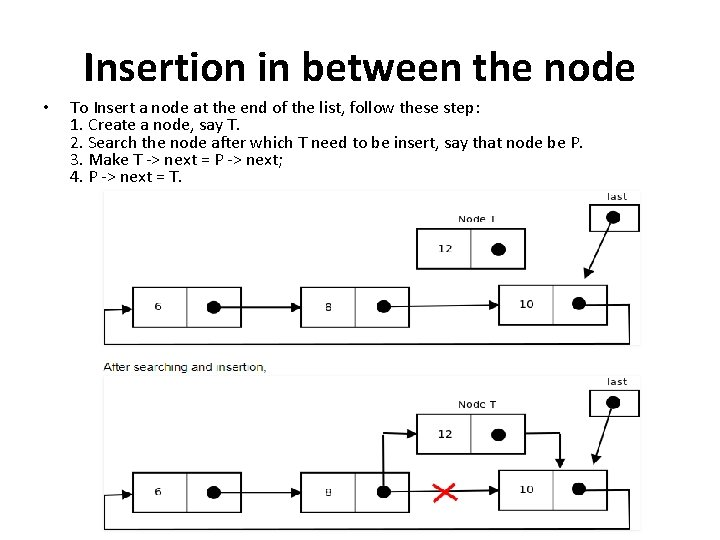Insertion in between the node • To Insert a node at the end of the list, follow these step: 1. Create a node, say T. 2. Search the node after which T need to be insert, say that node be P. 3. Make T -> next = P -> next; 4. P -> next = T.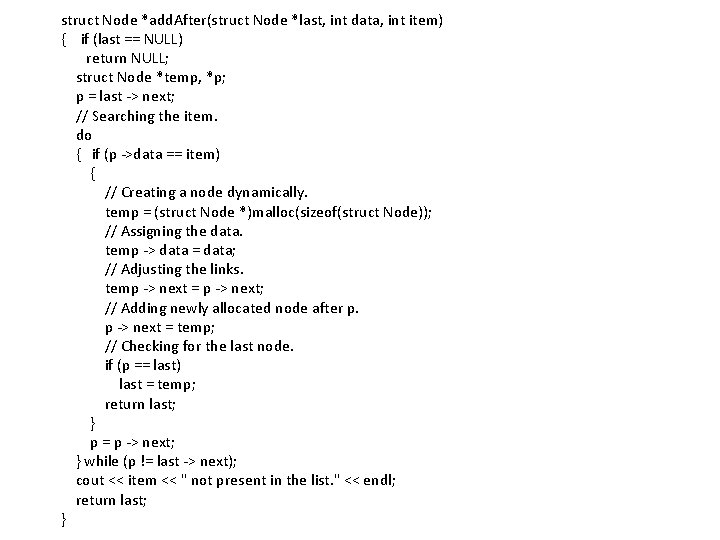struct Node *add. After(struct Node *last, int data, int item) { if (last == NULL) return NULL; struct Node *temp, *p; p = last -> next; // Searching the item. do { if (p ->data == item) { // Creating a node dynamically. temp = (struct Node *)malloc(sizeof(struct Node)); // Assigning the data. temp -> data = data; // Adjusting the links. temp -> next = p -> next; // Adding newly allocated node after p. p -> next = temp; // Checking for the last node. if (p == last) last = temp; return last; } p = p -> next; } while (p != last -> next); cout << item << " not present in the list. " << endl; return last; }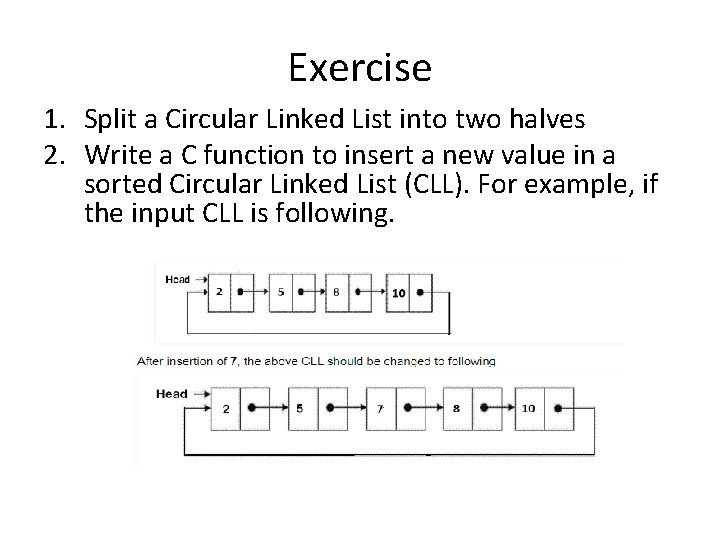Exercise 1. Split a Circular Linked List into two halves 2. Write a C function to insert a new value in a sorted Circular Linked List (CLL). For example, if the input CLL is following.